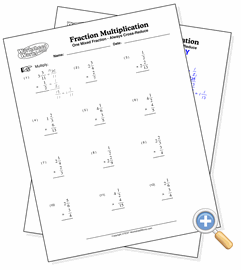# With One Mixed Fraction

## Fraction MultiplicationDevelop skills multiplying two mixed fractions

These problems involve the multiplication of a fraction and one mixed fraction. This provides the most difficult fraction multiplication problem since it involves converting to improper fractions, cross-reducing, multiplying and finally simplifying in each problem.